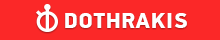GMAT Question of the Day - Daily to your Mailbox; hard ones only

 It is currently 26 Jun 2019, 11:36GMAT Club Daily Prep

Thank you for using the timer - this advanced tool can estimate your performance and suggest more practice questions. We have subscribed you to Daily Prep Questions via email.

Customized
for You

we will pick new questions that match your level based on your Timer History

Track

every week, we’ll send you an estimated GMAT score based on your performance

Practice
Pays

we will pick new questions that match your level based on your Timer History

Not interested in getting valuable practice questions and articles delivered to your email? No problem, unsubscribe here.The perimeter of a rectangle is 6w. If one side w as length w/2 what

Author Message
TAGS:

Hide Tags

Math ExpertV
Joined: 02 Sep 2009
Posts: 55803
The perimeter of a rectangle is 6w. If one side w as length w/2 what  [#permalink]

Show Tags00:00

Difficulty:45% (medium)

Question Stats:67% (01:30) correct33% (01:26) wrongbased on 22 sessions

HideShow timer StatisticsThe perimeter of a rectangle is 6w. If one side w as length w/2 what is the area of the rectangle?

A. w^2/4

B. 5w^2/4

C. 5w^2/2

D. 11w^2/4

E. 11w^2/2

_________________
InternB
Joined: 23 Feb 2018
Posts: 11
Location: India
GPA: 3
Re: The perimeter of a rectangle is 6w. If one side w as length w/2 what  [#permalink]

Show Tags

OA is B

2(l+b)=6w
so l+b=3w
if one of the sides is 1/2w then the longer side is 2.5w, when multiplied gives 2.5w^2/2, the only answer that matches the fractional value is B.

Bunuel wrote:
The perimeter of a rectangle is 6w. If one side w as length w/2 what is the area of the rectangle?

A. w^2/4

B. 5w^2/4

C. 5w^2/2

D. 11w^2/4

E. 11w^2/2

_________________
“The man who asks a question is a fool for a minute, the man who does not ask is a fool for life.”
CEOP
Joined: 18 Aug 2017
Posts: 3947
Location: India
Concentration: Sustainability, Marketing
GPA: 4
WE: Marketing (Energy and Utilities)
Re: The perimeter of a rectangle is 6w. If one side w as length w/2 what  [#permalink]

Show Tags

Bunuel wrote:
The perimeter of a rectangle is 6w. If one side w as length w/2 what is the area of the rectangle?

A. w^2/4

B. 5w^2/4

C. 5w^2/2

D. 11w^2/4

E. 11w^2/2

2 * (l+b) = w
b = 5w/2 ; l= w/2

area ; 5 w^2/4
IMO B
_________________
If you liked my solution then please give Kudos. Kudos encourage active discussions.
VPG
Joined: 09 Mar 2018
Posts: 1003
Location: India
Re: The perimeter of a rectangle is 6w. If one side w as length w/2 what  [#permalink]

Show Tags

Bunuel wrote:
The perimeter of a rectangle is 6w. If one side w as length w/2 what is the area of the rectangle?

A. w^2/4

B. 5w^2/4

C. 5w^2/2

D. 11w^2/4

E. 11w^2/2

2(l+b) = 6w
l+b = 3w

l=w/2

b will be now 5w/ 2

Area of a rectangle = l * b
=>$$\frac{5w^2}{4}$$
_________________
If you notice any discrepancy in my reasoning, please let me know. Lets improve together.

Quote which i can relate to.
Many of life's failures happen with people who do not realize how close they were to success when they gave up.Re: The perimeter of a rectangle is 6w. If one side w as length w/2 what   [#permalink] 11 Jan 2019, 04:11
Display posts from previous: Sort by

The perimeter of a rectangle is 6w. If one side w as length w/2 what